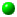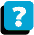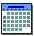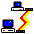Module 4.6 Notes "Comparing Multiple Samples: A Nonparametric Test"

 Index to Module 4 Notes4.1 Comparing 2 Independent Samples of Numerical Data4.2 Comparing 2 Related Samples of Numerical Data4.3 Comparing 2 Samples Using a Nonparametric Test4.4 Comparing Multiple Samples: One Factor4.5 Comparing Multiple Samples: Two Factors4.6 Comparing Multiple Samples: A Nonparametric Test

Recall in Module Notes 4.3 we introduced the technique for comparing means of multiple samples using the One-Factor ANOVA model. The assumptions required for this model are normality and homogeneity of variance. When the assumptions of normality and homogeneity of variance are not met, especially with small samples, and/or especially when there is not an equal sample size in each group, a nonparametric alternative to the Single or One-Factor ANOVA is the Kruskal-Wallis Rank Test. This test is similar to the nonparametric test used as an alternative to the two independent group t-Test presented in Module Notes 4.3 in that it uses ranks of the observations rather than the observations themselves.

If nothing is known about the variability of the shape of the distributions from which the multiple group samples are drawn, then the hypotheses statements are (Aczel, p. 656):

H0: All of the multiple populations have the same distribution
Ha: Not all of the multiple populations have the same distribution

One of the reference texts (Levine) notes that if the populations are assumed to have the same variability and shape, then location hypotheses can be stated as:

H0: All of the population medians are equal
Ha: At least two of the medians are not equal

In either case, we also assume that the samples are randomly and independently drawn from the respective populations.

Let's demonstrate the Kruskal-Wallis Rank Test with the miles per gallon example used in Module Notes 4.3. Worksheet 4.6.1 provides the sorted mpg data for the seven automobiles randomly and independently assigned to Brand of gas Groups A, B and C.
Worksheet 4.6.1

 Brand A Rank A Brand B Rank B Brand C Rank C 14 1 15 2 16 3 17 4 18 5 18.5 6 19 8 19 8 19 8 20 12 20 12 20 12 20 12 20 12 20.5 15 23 17 23 17 23 17 24 19 25 20 26 21 Rank Sum 42 66 123 TA TB TC

Worksheet 4.6.1 also contains the rankings for the observations within each group, using the same ranking technique as in Module Notes 4.3.

A check on the rankings is provided by Equation 4.6.1. :

Eq. 4.6.1: TA + TB + TC = n ( n + 1 ) / 2

The sum of the ranks is 231 (42 + 66 +123). The total sample size, n, is 21 so the right hand side of Eq. 4.6.1 is 21 (21 + 1) / 2 which equals 231. Therefore, the rankings check.

The Kruskal-Wallis Test Statistics is shown in Eq. 4.6.2:

Eq. 4.6.2: H = [12/n(n+1)] * [TA2/n + TB2/n + TC2/n] - 3(n+1)
= {12/[21(21+1)]} * [422/7 + 662/7 + 1232/7] - 3 (21 + 1) = 12.846

This Test Statistic is compared to a critical or threshold value for the chi-square Statistic. If the Test Statistic is greater than the chi-square critical value, we reject the null hypothesis and conclude that the medians are not equal. I will present and discuss the chi-square Distribution in Module 5. For now, we will let Excel simply return the Chi Square Critical Value.

The Excel function for this is =CHIINV(alpha, degrees of freedom). We will use alpha of 0.05, and number of groups being compared minus one for the degrees of freedom. So, in an active cell in an Excel Worksheet, enter =CHIINV(0.05,3-1) and Excel will return 5.99. Since 12.846 is greater than 5.99, we reject the null hypothesis and conclude that the groups are different (the population distributions are different or the medians of the three groups are not equal).

Now, what do we do after we reject the null hypothesis? That is, we know that the three groups are not equal, but where do the differences lie? Recall in Module Notes 4.4, we introduced the Bonferroni Post Hoc procedure to determine which pairs of means were significantly different in single factor ANOVA. That's what we can do with the following post hoc procedure to the Kruskal Wallis Test.

Suppose we want to determine if the Brand A population was different from the Brand C population. First, find the average ranks of the A and C groups:

Eq. 4.6.3: Mean RankA = TA/7 = 42 / 7 = 6
Eq. 4.6.4: Mean RankC = TC/7 = 123/7 = 17.6

Now find the absolute difference between the two mean ranks:

Eq. 4.6.5: Difference = | 17.6 - 6 | = 11.6

Now find the critical point for this paired difference. If the paired difference is greater than the critical point, reject the null hypothesis of equality and conclude that the Brand A population distribution is different than the Brand C population distribution. The equation for the critical point is:

Eq. 4.6.6: CKW = Sq Rt{Chi Square*[n(n+1)/12]*[(1/nA)+(1/nB)]}
= Sq Rt{5.99*[21(21+1)/12]*[(1/7) + (1/7)]} = 8.11

Since 11.6 is greater than 8.11, we reject the null hypothesis and conclude that Brand A population distribution is different than the Brand C population distribution. If we have prior knowledge that the variability of the populations is the same, we may extend this conclusion to state that the population medians are not the same.

This ends Module 4: a set of tools to use for the comparison of multiple samples.

References:

Aczel, A. (1993). Complete Business Statistics (2nd. ed.). Homewood, IL: Irwin.

Levine, D., Berenson, M. & Stephan, D. (1999). Statistics for Managers Using Microsoft Excel (2nd. ed.). Upper Saddle River, NJ: Prentice-Hall, Chapter 10.

Mason, R., Lind, D. & Marchal, W. (1999). Statistical Techniques in Business and Economics (10th. ed.). Boston: Irwin McGraw Hill, Chapter 15.About the CourseModule ScheduleWebBoard